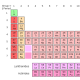Sunday, November 2, 2014 2

So, you are around two months into your IB Chemistry course. How is it going? Easy? Difficult? Indifferent?

To start with your teacher or teachers have probably taught you topic 1 (Stoichiometric Relationships) or topic 2 and 12 (Atomic Structure). It doesn’t really matter which was around they have taught you but I would be really surprised if they had started with something different.

Part of the HL atomic structure part of the course involves studying  ionization energies and how they provide evidence for shells and  sub-shells and also studying atomic emission spectra. Further to this the new course has cleverly gelled these two ideas together and you are also expected to be able to calculate ionization energies from emission spectra data.

The calculation can seem a little daunting as the numbers involved are both very large and very small – but don’t let this put you off. I have put together a set of steps that will (I hope!) help you understand this and successfully calculate the value.

1, Take the reciprocal of the wavelength (ie, put 1 over the value)

2, This will give you a wavelength in cm, you need to convert the value into m so divide by 100.

3, Calculate the frequency using c = λ

* c = speed of light (you can get this from the data book). The units of v are s-1

4, Calculate the energy to remove this single electron using E = hv (h = Planck’s constant – see data book again). This will give you the energy to remove one electron in Joules.

5, Multiply the value by 6.02 x 10 23 (ie, Avagadro’s constant) to get the value in J mol -1

6, Finally, divide by 1000 to get the answer in KJ mol -1.

Phew!

Did you understand this? Do you have any further questions?

Try this question to test your understanding:

Question: Given that the wavelength of convergence for Vanadium is 54411.7 cm -1, calculate the first ionisation energy for the element.

Please post your answer below and I’ll let you know if it is correct – I need to see your working out though – don’t go cheating and looking it up in the data book!

Next month my intention is to pick up on some other ‘new’ areas of the course that you will have been taught / will be taught very soon.

•January 5, 2017

Hi so this is for when you get given the wavelength of the convergence, would you simply go from step 4 if you were given the frequency of convergence or would you need to do something different?

•January 9, 2017

Hi Haz,
Tat sounds correct to me. Have you been given this task as a question?

•January 26, 2017

When looking at an emission spectrum with many lines of various wavelengths, how do I find the ionization energy? Do I take the sum of each number I get or something similar?

•February 6, 2017

Hi Eoin,
Thanks for the question.
In order to answer this you would need to identify the n=1 (or whatever is the lowest energy level of the outermost electron) to n=infinity transition wavelength from the spectra – easier said than done! For hydrogen, it will be in the Lyman series (as this deals with transitions from n=1) and will have the lowest wavelength.
You really need to be given the wavelength in order to answer the question, as far as I know you can’t do it from just looking at the spectra.
But I stand to be corrected if anyone else can help!
Dave

•March 14, 2018

Hi,

Is the answer: 2.20 * 10^-7 KJ/mol

•March 17, 2018

•June 26, 2019

How do you express wavelength in cm-1?. is it wave number?

E =hc/lambda gives IE in joules for an atom

multiply the value with plank constant and divide with 1000 gives IE in KJ/mol

•July 3, 2019

Hi Siji, thanks for the message. Wavenumber is 1/wavelength. Hope that helps! Dave